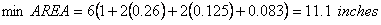# FWD AREA Parameter

FWD tests produce a series of numbers that describes the deflection basin shape.  Although these numbers are descriptive, it is often desired to use a single number as a index of the deflection basin shape.  One such number, the AREA Parameter represents the normalized area of a vertical slice taken through a deflection basin between the center of the test load and 914 mm (3 feet) away from the test load.  Dividing the slice area by the deflection measured at the center of the test “normalizes” the AREA Parameter.  Thus the AREA Parameter is the length of one side of a rectangle where the other side of the rectangle is D0.

The basic AREA Parameter equation is:where: AREA equals the FWD AREA Parameter. Expressed in units of length (usually inches or mm). D0 equals surface deflection at the test load center D1 equals surface deflection at 12 inches from the test load center D2 equals surface deflection at 24 inches from the test load center D3 equals surface deflection at 36 inches from the test load center

## Maximum and Minimum AREA Values

The maximum AREA value is 36 inches (915 mm) and would occur when all four deflection measurements are equal, which is not likely to actually occur.  For all four deflection measurements to be nearly equal the pavement would have to be extremely stiff (like rigid pavement or thick, full-depth HMA).  The equation for the maximum AREA value is as follows:The minimum Area value should be no less than 11.1 in. (280 mm) and would occur when all pavement layers have the same elastic modulus.  Or, put another way, the pavement structure does not contribute any additional stiffness to the underlying subgrade.  This is highly unlikely for actual pavement structures.  Low AREA values suggest that the pavement structure is not much different from the underlying subgrade material.  This is not always a bad thing if the subgrade is extremely stiff, but this does not occur very often).  When all elastic moduli are equal the ratio of D1, D2 and D3 to D0 are as follows, which gives the minimum AREA value as shown below:## Typical AREA Values

Table 1 shows some typical AREA values.

 Pavement AREA Value inches mm Rigid pavement 24 – 33 610 – 840 Thick flexible pavement >= 100 mm (4 inches) 21 – 30 530 – 760 Thin flexible pavement < 100 mm (4 inches) 16 – 21 410 – 530 BST 15 – 17 380 – 430 Weak BST 12 – 15 300 – 380

## FWD Area Program

WSDOT has developed a program for the determination of the AREA Value and subgrade resilient modulus using the Dynatest FWD.

#### NOTES:

• This program must be installed on to your computer before you can use it. During the installation you will be prompted to specify a location to which it can be installed. Once installed, the program and its supporting files take about 450 kB of disk space.
• The program is designed for Windows operating systems.

The FWD Area program can also be downloaded from the Washington State DOT Materials Lab at: http://www.wsdot.wa.gov/biz/mats.